The Golden Ratio

Euclides, in his book The Elements, defines a proportion derived from a division of a segment into its "extreme and mean ratio".

"A straight line is said to have been cut in extreme and mean ratio when ,as the whole line is to the greater segment, so is the greater to the less." (Book IV, Definition 3)

Now we call this ratio the golden section, the golden ratio or the divine proportion. It is usually denoted by the greek letterphi, the initial letter of Phidias's name.

Euclid's construction for the regular pentagon depends on this ratio. Two crossing diagonals of a regular pentagon divide one another in extreme and mean ratio.

Using a strip of paper we can made a knot and get a pentagon and a pentagram.We also find the golden ratio in the dodecahedron and the icosahedron.

A rectangle is called a golden rectangle if it has its sides in the golden ratio. If we cut a golden rectangle into a square and a small rectangle this small rectangle is a golden rectangle. We can continue this process indefinitely.

We can deduce the value of the golden ratio.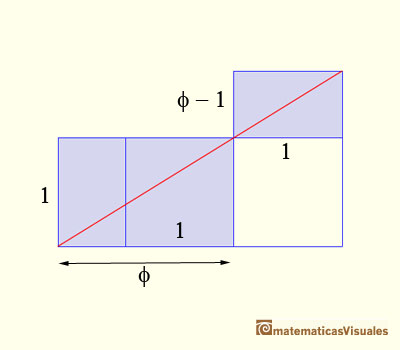The big rectangle and the small one are similar. We can write the ratio:

Clearing denominators we get a second degree equation:

The positive solution of this equation is the golden ratio:

REFERENCES

Euclides, The Elements
Coxeter - Introduction to Geometry (John Whiley and sons) pp. 160-163The diagonal of a regular pentagon are in golden ratio to its sides and the point of intersection of two diagonals of a regular pentagon are said to divide each other in the golden ratio or 'in extreme and mean ratio'.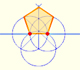In his book 'Underweysung der Messung' Durer draw a non-regular pentagon with ruler and a fixed compass. It is a simple construction and a very good approximation of a regular pentagon.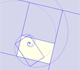A golden rectangle is made of an square an another golden rectangle. These rectangles are related through an dilative rotation.The golden spiral is a good approximation of an equiangular spiral.Two equiangular spirals contains all vertices of golden rectangles.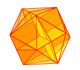The twelve vertices of an icosahedron lie in three golden rectangles. Then we can calculate the volume of an icosahedron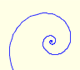In an equiangular spiral the angle between the position vector and the tangent is constant.Two transformations of an equiangular spiral with the same general efect.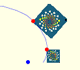A Dilative Rotation is a combination of a rotation an a dilatation from the same point.Some properties of this platonic solid and how it is related to the golden ratio. Constructing dodecahedra using different techniques.He studied transformations of images, for example, faces.The first drawing of a plane net of a regular dodecahedron was published by Dürer in his book 'Underweysung der Messung' ('Four Books of Measurement'), published in 1525 .One eighth of a regular dodecahedon of edge 2 has the same volume as a dodecahedron of edge 1.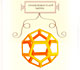Leonardo da Vinci made several drawings of polyhedra for Luca Pacioli's book 'De divina proportione'. Here we can see an adaptation of the dodecahedron.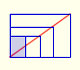There is a standarization of the size of the paper that is called DIN A. Successive paper sizes in the series A1, A2, A3, A4, and so forth, are defined by halving the preceding paper size along the larger dimension.